Ergodicity.

I recently came across a great explainer video on ergodicity by Alex Adamou on Ergodicity.tv

Ergodicity is an incredibly important concept to understand when dealing with multiple trajectories of a stochastic process -- which is simply a random process through time. Common examples of stochastic processes include games of chance (i.e. rolling a dice) and random walks (i.e. path a molecule takes as it travels in a liquid or gas).

Taking some examples from Alex, below is a graph representing a stochastic process X(t) with a set of four trajectories {$x_n(t)$}.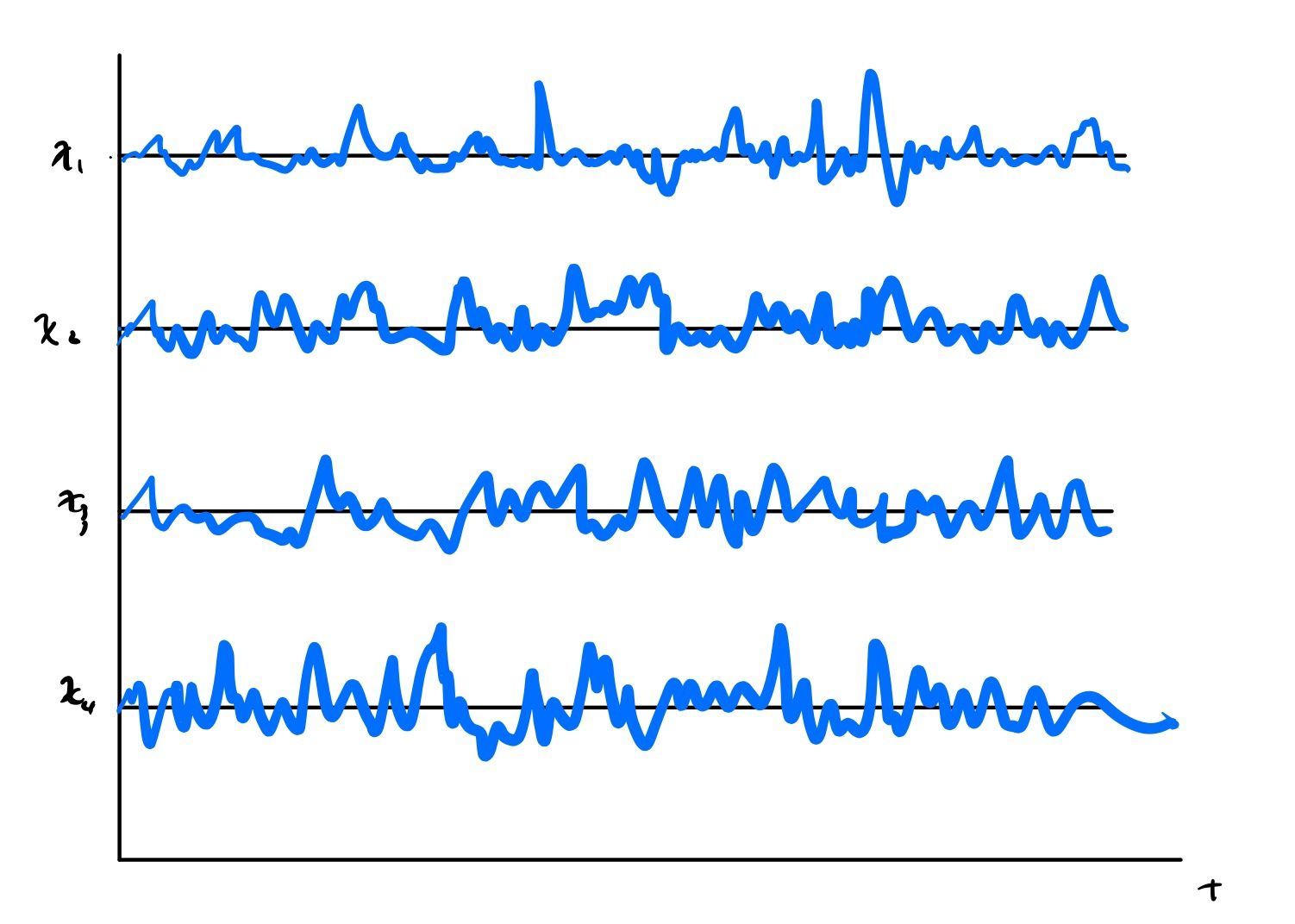To determine the finite time average we fix the trajectory and average horizontally over the time rangeWhen time diverges the time average is given by: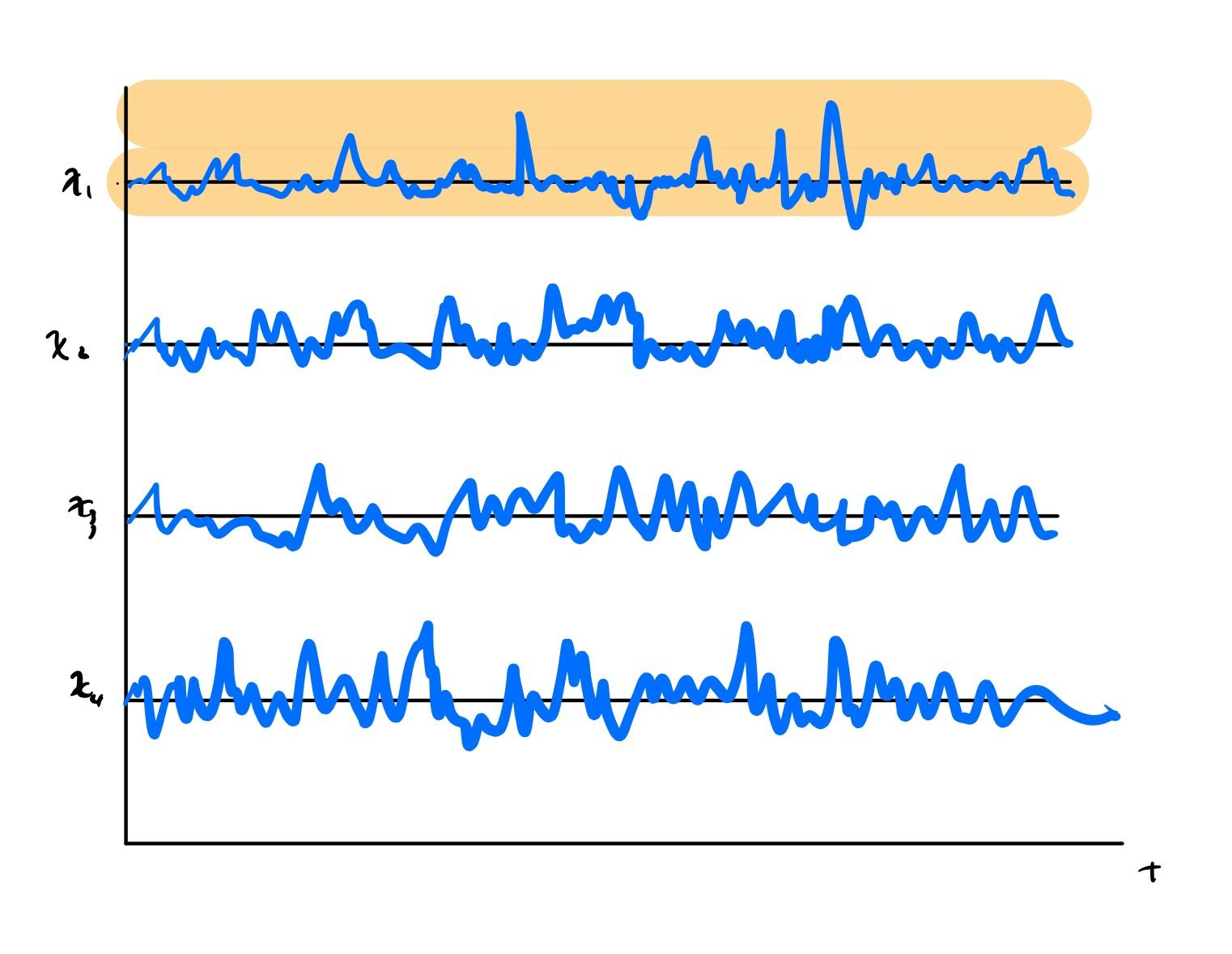In the finite ensemble average we fix time and average over the trajectories: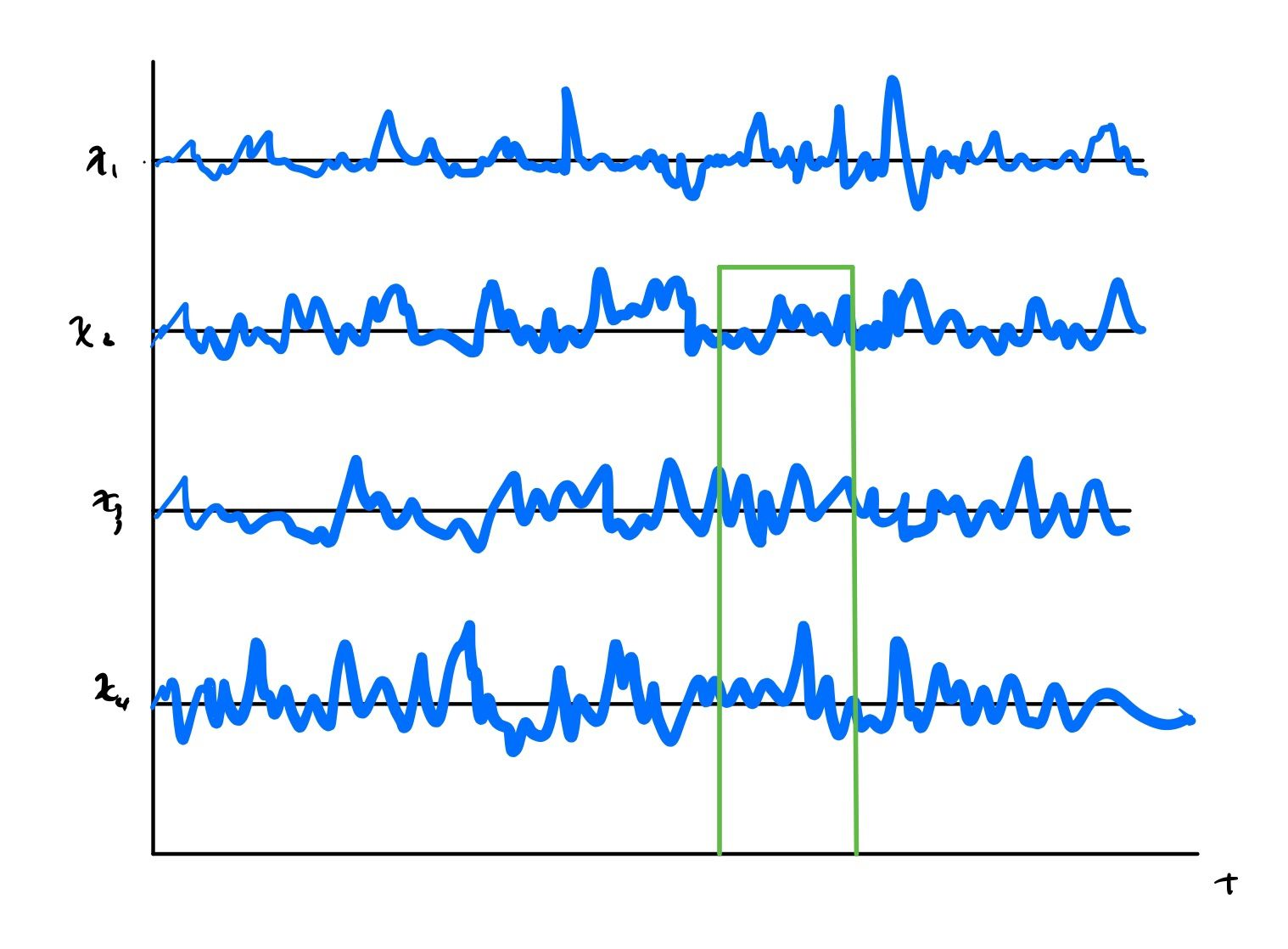When the number of systems diverge the ensemble average is given by: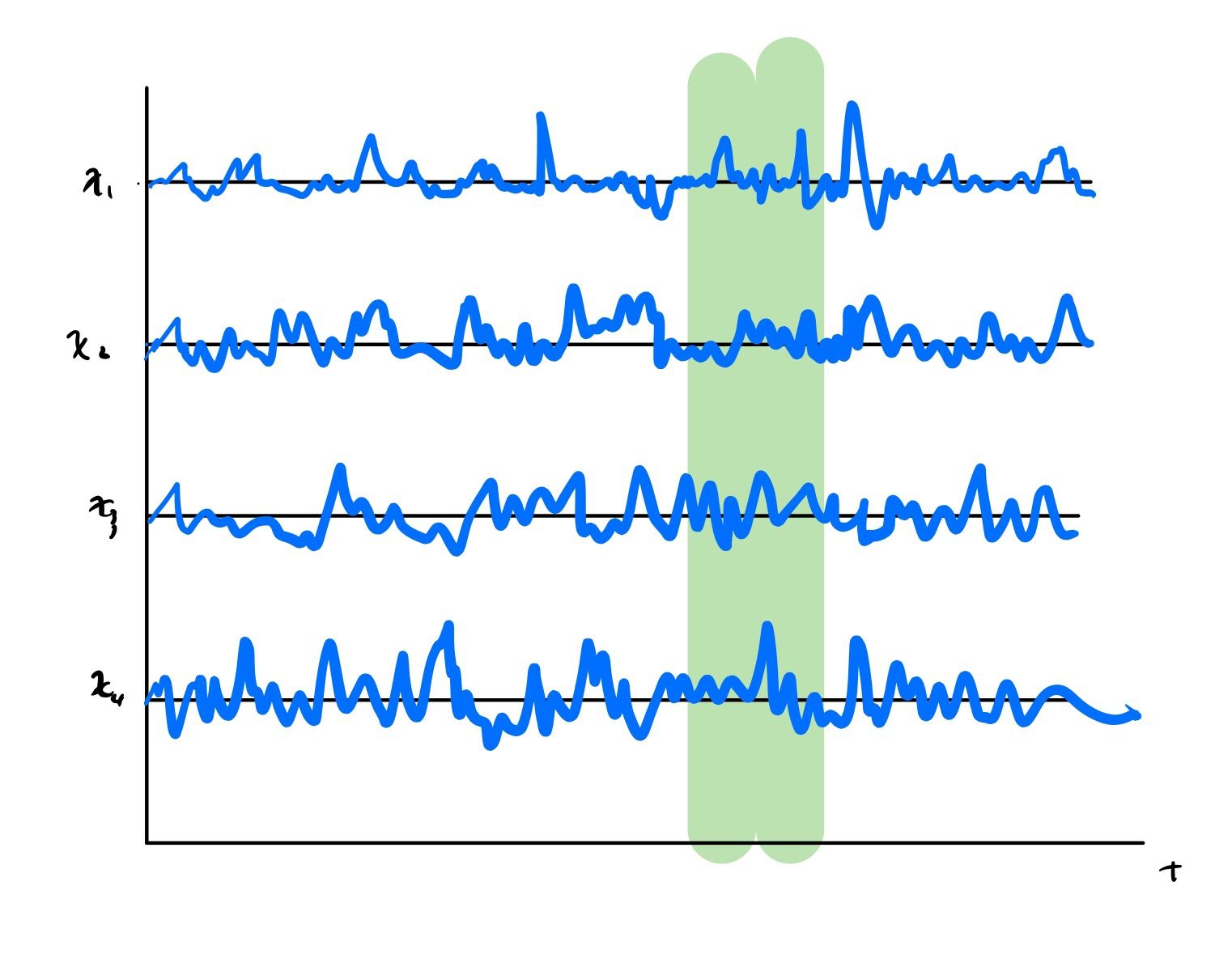A stochastic process is ergodic if the time average of a single trajectory is equal to the ensemble average.

Shown graphically, here is what we are examining: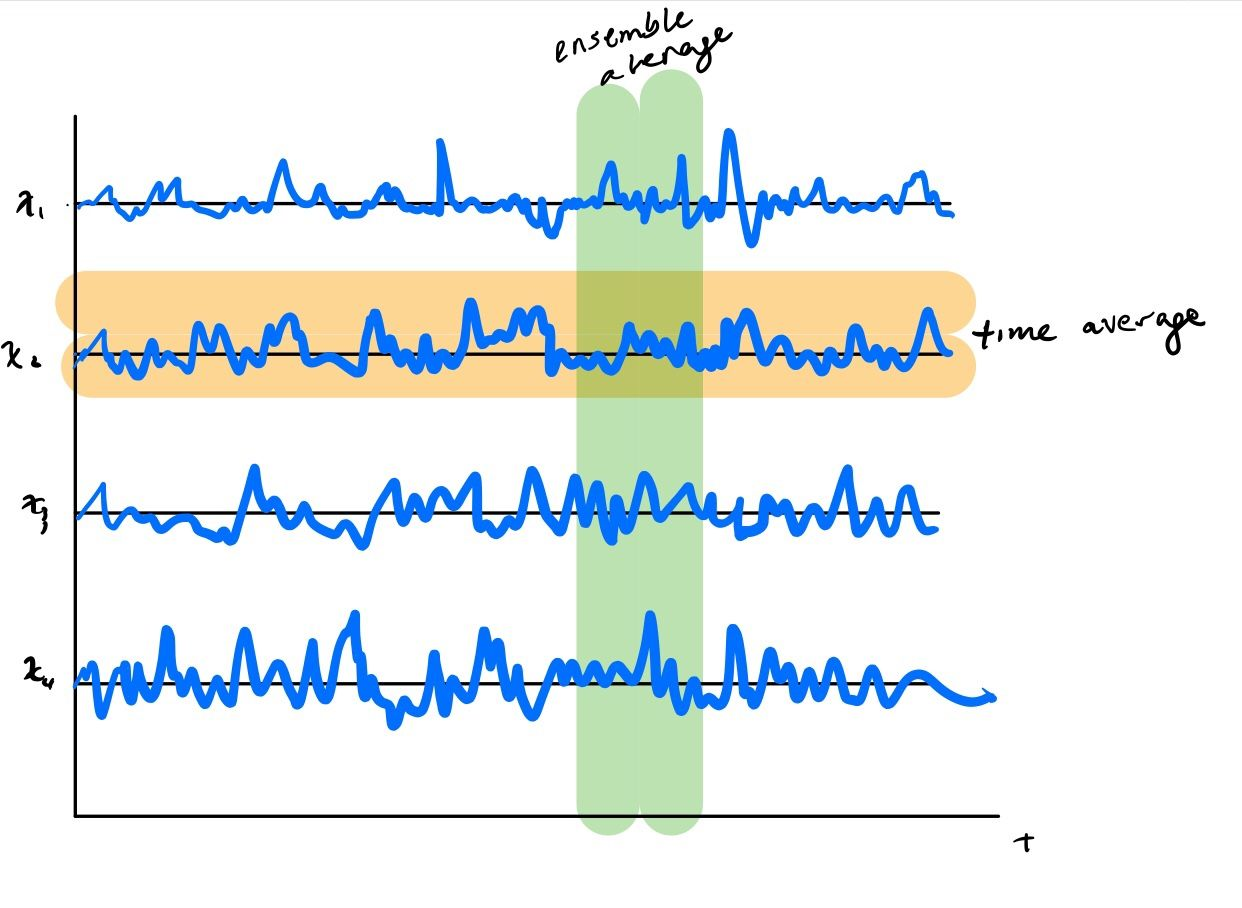And that’s really all there is to ergodicity! Ergodicity is a special case where the time average is equal to the time average.

Said differently, the time average and the ensemble average are only interchangeable when the process under consideration is ergodic.

This is a key point because the ensemble average is often easier to determine than the time average. Alex points to economics as an example where people often utilize expectation values to represent temporal phenomena without knowing if the system is ergodic.

In many real world scenarios, things exhibit strong path dependence meaning that they are very likely non-ergodic. The classic example is Polya’s urn where a ball is selected from an urn with balls of two different colors. The ball is then replaced and another ball of the same color that was selected is added to the urn. The first ball selected has a large impact on the subsequent makeup of the urn over multiple trials and breaks ergodicity.

I highly recommend watching Alex’ video for additional examples. I think he did a really good job of distilling the essence of ergodicity for the layman.